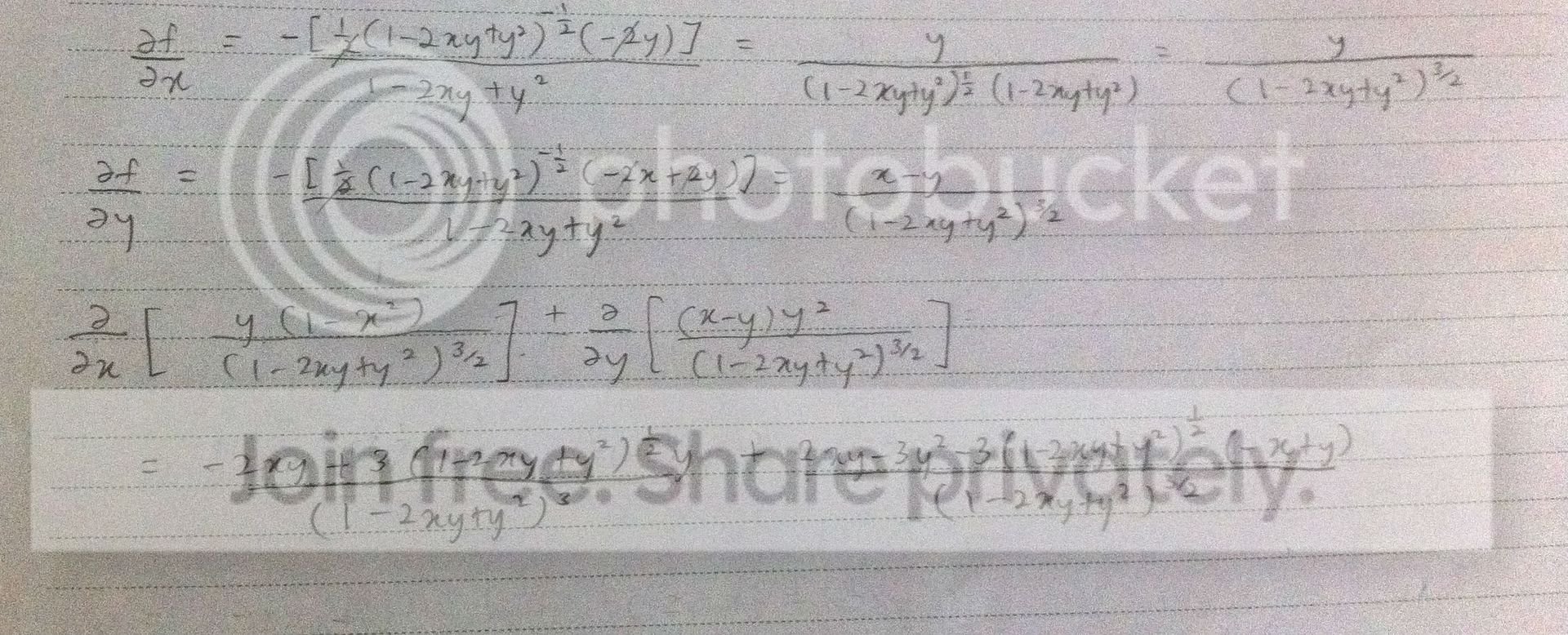# Please check my partial diferentiation

#### aruwin

##### Member
I have calculated 3 times and I still don't get the answer. The answer should be 0.
Here's the question and my work. Which part am I wrong?

f(x,y) = 1/√(1-2xy+y^2)

Prove that ∂/∂x{(1-x^2)*∂f/∂x} + ∂/∂y{(y^2)*∂f/∂y} = 0#### Sudharaka

##### Well-known member
MHB Math Helper
I have calculated 3 times and I still don't get the answer. The answer should be 0.
Here's the question and my work. Which part am I wrong?

f(x,y) = 1/√(1-2xy+y^2)

Prove that ∂/∂x{(1-x^2)*∂f/∂x} + ∂/∂y{(y^2)*∂f/∂y} = 0Hi aruwin,You have used the quotient rule incorrectly when calculating, $$\displaystyle\frac{\partial}{\partial x}\left[\frac{y(1-x^2)}{(1-2xy+y^2)^{\frac{3}{2}}}\right]\mbox{ and }\frac{\partial}{\partial y}\left[\frac{(x-y)y^2}{(1-2xy+y^2)^{\frac{3}{2}}}\right]$$.

Kind Regards,
Sudharaka

•Jameson

#### aruwin

##### Member
Hi aruwin,You have used the quotient rule incorrectly when calculating, $$\displaystyle\frac{\partial}{\partial x}\left[\frac{y(1-x^2)}{(1-2xy+y^2)^{\frac{3}{2}}}\right]\mbox{ and }\frac{\partial}{\partial y}\left[\frac{(x-y)y^2}{(1-2xy+y^2)^{\frac{3}{2}}}\right]$$.

Kind Regards,

Sudharaka
Oh my gosh, yes. I have found what is wrongLast edited by a moderator:

#### Fantini

Let's try using the product rule: $$\frac{\partial}{\partial x} \left[ (y-x^2y)(1-2xy+y^2)^{-\frac{3}{2}} \right]$$ $$= (-2xy)(1-2xy+y^2)^{-\frac{3}{2}} + (y-x^2y)\left( - \frac{3}{2} \right) (-2y) (1-2xy+y^2)^{-\frac{5}{2}}$$ $$= \frac{(-2xy)(1-2xy+y^2) + (y-x^2y)(3y)}{(1-2xy+y^2)^{\frac{5}{2}}}$$ $$= \frac{-2xy+4x^2y^2-2xy^3+3y^2-3x^2y^2}{(1-2xy+y^2)^{\frac{5}{2}}}$$ $$= \frac{-2xy+3y^2-2xy^3+x^2y^2}{(1-2xy+y^2)^{\frac{5}{2}}}.$$ Hope it helps.
•Thanks,guys!I have solved this question